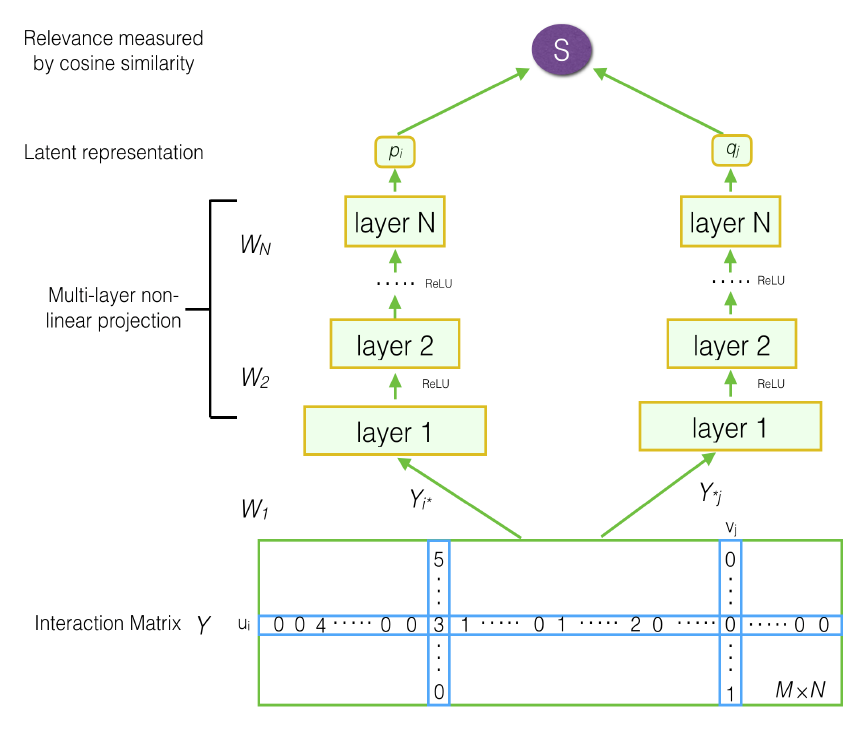DMF¶

Introduction¶

[paper]

Title: Deep Matrix Factorization Models for Recommender Systems

Authors: Hong-Jian Xue, Xin-Yu Dai, Jianbing Zhang, Shujian Huang, Jiajun Chen

Abstract: Recommender systems usually make personalized recommendation with user-item interaction ratings, implicit feedback and auxiliary information. Matrix factorization is the basic idea to predict a personalized ranking over a set of items for an individual user with the similarities among users and items. In this paper, we propose a novel matrix factorization model with neural network architecture. Firstly, we construct a user-item matrix with explicit ratings and non-preference implicit feedback. With this matrix as the input, we present a deep structure learning architecture to learn a common low dimensional space for the representations of users and items. Secondly, we design a new loss function based on binary cross entropy, in which we consider both explicit ratings and implicit feedback for a better optimization. The experimental results show the effectiveness of both our proposed model and the loss function. On several benchmark datasets, our model outperformed other state-of-the-art methods. We also conduct extensive experiments to evaluate the performance within different experimental settings.Running with RecBole¶

Model Hyper-Parameters:

• user_embedding_size (int) : The initial embedding size of users. Defaults to 64.

• item_embedding_size (int) : The initial embedding size of items. Defaults to 64.

• user_hidden_size_list (list) : The hidden size of each layer in MLP for users, the length of list is equal to the number of layers. Defaults to [64,64].

• item_hidden_size_list (list) : The hidden size of each layer in MLP for items, the length of list is equal to the number of layers. Defaults to [64,64].

• inter_matrix_type (str) : Use the implicit interaction matrix or the rating matrix. Defaults to '01'. Range in ['01', 'rating'].

A Running Example:

Write the following code to a python file, such as run.py

from recbole.quick_start import run_recbole

run_recbole(model='DMF', dataset='ml-100k')

And then:

python run.py

Notes:

• The last value in user_hidden_size_list and item_hidden_size_list must be the same.

• If you set inter_matrix_type='rating', the ‘rating’ field from *.inter atomic files must be remained which means that the rating cannot be included in unload_col or unused_col.

Tuning Hyper Parameters¶

If you want to use HyperTuning to tune hyper parameters of this model, you can copy the following settings and name it as hyper.test.

learning_rate choice [0.01,0.005,0.001,0.0005,0.0001]
user_layers_dim choice ['[64, 64]','[64, 32]','[128,64']]
item_layers_dim choice ['[64, 64]','[64, 32]','[128,64']]

Note that we just provide these hyper parameter ranges for reference only, and we can not guarantee that they are the optimal range of this model.

Then, with the source code of RecBole (you can download it from GitHub), you can run the run_hyper.py to tuning:

python run_hyper.py --model=[model_name] --dataset=[dataset_name] --config_files=[config_files_path] --params_file=hyper.test

For more details about Parameter Tuning, refer to Parameter Tuning.

If you want to change parameters, dataset or evaluation settings, take a look at